# 9.3: Nomenclature and Ligands

$$\newcommand{\vecs}{\overset { \rightharpoonup} {\mathbf{#1}} }$$ $$\newcommand{\vecd}{\overset{-\!-\!\rightharpoonup}{\vphantom{a}\smash {#1}}}$$$$\newcommand{\id}{\mathrm{id}}$$ $$\newcommand{\Span}{\mathrm{span}}$$ $$\newcommand{\kernel}{\mathrm{null}\,}$$ $$\newcommand{\range}{\mathrm{range}\,}$$ $$\newcommand{\RealPart}{\mathrm{Re}}$$ $$\newcommand{\ImaginaryPart}{\mathrm{Im}}$$ $$\newcommand{\Argument}{\mathrm{Arg}}$$ $$\newcommand{\norm}{\| #1 \|}$$ $$\newcommand{\inner}{\langle #1, #2 \rangle}$$ $$\newcommand{\Span}{\mathrm{span}}$$ $$\newcommand{\id}{\mathrm{id}}$$ $$\newcommand{\Span}{\mathrm{span}}$$ $$\newcommand{\kernel}{\mathrm{null}\,}$$ $$\newcommand{\range}{\mathrm{range}\,}$$ $$\newcommand{\RealPart}{\mathrm{Re}}$$ $$\newcommand{\ImaginaryPart}{\mathrm{Im}}$$ $$\newcommand{\Argument}{\mathrm{Arg}}$$ $$\newcommand{\norm}{\| #1 \|}$$ $$\newcommand{\inner}{\langle #1, #2 \rangle}$$ $$\newcommand{\Span}{\mathrm{span}}$$$$\newcommand{\AA}{\unicode[.8,0]{x212B}}$$

## Systems of nomenclature and formulae are intended as tools, to be employed insofar as they are useful

There are well-established rules for both naming and writing the formulae of coordination compounds. The purpose of these rules is to facilitate clear and precise communication among chemists. As with all such rules, some are more burdensome than others to employ, and some serve more crucial roles in the communication process while others are more peripheral - and all are poorly used in the service of pedantic tyranny, especially when used against those who are otherwise doing good work. For this reason, you are urged to approach the rules in a spirit of generosity towards others in

• naming and writing formulas as reasonably accurately as you can so that ligands and metals are where readers expect them and thus can understand what you mean more easily.
• being gracious towards the many professional inorganic chemists who adhere loosely to some of the rules you are about to learn.
• recognizing that in cases when a structure is particularly complex and a picture may make be particularly useful, you should supply one (See the note below).
##### Note $$\sf{\PageIndex{1}}$$: Sometimes the most helpful name to give a compound is 42.

Even though the IUPAC nomenclature rules permit specification of even the most complex structures, it is often much easier and more effective to supply a numbered structure that can be referred to instead of the IUPAC name. Consider bis{[($$\mu$$-2-mercaptoethyl)(2-mercaptoethyl)-methylthioethylaminato (2-)]Nickel(II)}. Which is easier, to expect readers and hearers to work out the structure from that name or to just refer them to compound 42 in Scheme $$\sf{\PageIndex{I}}$$?Scheme $$\sf{\PageIndex{I}}$$. Structure of bis{[($$\mu$$-2-mercaptoethyl)(2-mercaptoethyl)-methylthioethylaminato (2-)]Nickel(II)}. The authors of the synthesis of this compound in Inorganic syntheses1 may have had to figure out an IUPAC name for this compound but if you have this scheme in your paper and your instructor is OK with it you can just call it 42

## Coordination Complexes are named as the ligand derivatives of a metal

A variety of systems have been used for naming coordination compounds since the development of the discipline in the time of Alfred Werner. In this section the most common approaches as they are currently used by practicing chemists will be described. Those who need a more thorough and accurate acquaintance with the full IUPAC nomenclature rules are encouraged to consult the IUPAC brief guide to inorganic nomenclature followed by complete guidelines, commonly known as the IUPAC red book. If those are still not enough a careful read of note $$\sf{\PageIndex{1}}$$ is suggested.

The systems for naming coordination compounds used at present are additive, meaning that they consider coordination compounds as comprising a central metal to which are added ligands. To specify the structure and bonding in this metal-ligand complex then involves:

1. when there are several different ways of attaching the metal and ligands, specifying the structural or stereoisomer
2. systematically listing the ligands in a way that, as necessary, conveys information about how they are linked to the metal and their stereochemistry
3. providing the identity of the metal and its oxidation state, or if the oxidation state is unclear, at least the overall charge on the complex
4. specifying any counterions present

Since the stereochemistry of coordination compounds forms the subject of the next section, in this section it will be addressed by simply giving the prefixes that designate stereochemistry as if they were self-evident. Do not worry about these for now. They will make sense after you have learned more about stereochemistry in the next section. At that time, you can go back over the examples in that section to solidify your understanding of how to name coordination compounds.

However, in order to name coordination compounds accurately you will need to learn how to think about and name ligands first.

## Ligands

Ligands are classified based on whether they bind to the metal center through a single site on the ligand or whether they bind at multiple sites. Ligands that bind through only a single site are called monodentate from the Latin word for tooth; in contrast, those which bind through multiple sites are called chelating after the Greek $$\chi \alpha \lambda \epsilon$$ for “claw”. These relationships are summarized in Figure $$\sf{\PageIndex{1}}$$.Figure $$\sf{\PageIndex{1}}$$. (A) Ammonia is a monodentate ligand while (B) ethylene diamine is a chelating ligand owing to its capacity to bind metals via its two amine functional groups. (C) Chelating ligands act like a lobster claw in attaching to the metal via multiple sites. The lobster claw image is adapted from https://www.clipart.email/download/1127636.html. Otherwise this work by Stephen Contakes is licensed under a Creative Commons Attribution 4.0 International License.

Following naturally from the classification of non-chelating ligands as monodentate, chelating ligands are further classified according to the number of sites which they can use to bind a metal center. This number of binding sites is called the denticity and ligands are referred to as monodentate (non- chelating), bidentate, tridentate, etc., based on the number of sites available. Ligands with two binding sites have a denticity of two and are said to be bidentate; those with three are tridentate, four tetradentate, and so on. To illustrate this classification system examples of chelating ligands classified according to denticity are given in Scheme $$\sf{\PageIndex{I}}$$.

Scheme $$\sf{\PageIndex{I}}$$. A selection of chelating ligands classified according to denticity.Scheme $$\sf{\PageIndex{IIA}}$$. Because only one oxygen per carboxylate typically binds, only one is counted when assigning a ligand's denticity. This work by Stephen Contakes is licensed under a Creative Commons Attribution 4.0 International License.

Although only one carboxylate oxygen usually binds to a metal, it is still possible to bind a metal using both oxygens. As shown in Scheme $$\sf{\PageIndex{IIB}}$$, complexes in which both carboxylates bind to a metal are known, and in fact are common in the active sites of some enzymes. It is just that the binding of both oxygens gives a strained four-membered ring that is usually unstable.

Scheme $$\sf{\PageIndex{II}}$$. (A) Only one oxygen per carboxylate counts towards the denticity of EDTA since on binding the other oxygen generally points away from the metal center, as in the structure of Fe(EDTA)-. This does not mean that both oxygens of a carboxylate can never both bind to metal centers in a complex. (B) Structures in which both oxygens of a carboxylate side chain bind to a metal are sometimes found in the active sites of some of the nonheme iron enzymes your body uses to break down amino acids.Scheme $$\sf{\PageIndex{III}}$$) Two atoms from one ligand bind and are classified as bidentate. This work by Stephen Contakes is licensed under a Creative Commons Attribution 4.0 International License.

Scheme $$\sf{\PageIndex{III}}$$. As in this complex, dithiocarbamates commonly bind metals through both sulfur atoms. Consequently, dithiocarbamates are classified as bidentate. This work by Stephen Contakes is licensed under a Creative Commons Attribution 4.0 International License.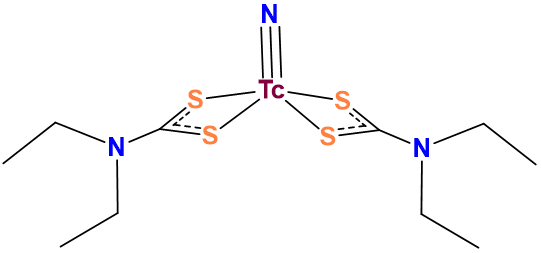Because of these factors it is technically more correct to say that carboxylates usually act as monodentate ligands and dithiocarbamates bidentate ones than it is to say that carboxylates are monodentate ligands and dithiocarbamates bidentate ones. So in other words the ligand classifications presented here just represent common binding modes.

##### Exercise $$\PageIndex{1}$$

Determine the denticity of each ligand in the list below and classify them as monodentate, tridentate, etc.(a) bidentate

(b) tridentate

(c) bidentate

(d) tridentate (only the lower N on each ring has a lone pair that can be used to bind the metal)

(e) hexadentate (remember that each carboxylate only counts as one point of attachment)

(f) bidentate

(g) monodentate (through the lone pair on the isocyanide C)

(h) bidentate

This experimentally-based classification of dithiocarbamates as bidentate and carboxylates as monodentate can be confusing to a beginner. Fortunately, such experimentally based classifications are embedded in the lists of common monodentate ligands as given in Table $$\sf{\PageIndex{1}}$$ and common chelating ligands in Table $$\sf{\PageIndex{2}}$$.

A perusal of the ligands in Table $$\sf{\PageIndex{1}}$$ reveals that several can bind to a metal in multiple ways. For example, thiocyanate, SCN- can bind metals through its S or N atoms. Such ligands are called ambidentate ligands. In naming an ambidentate ligand, the atom through which it attaches to the metal is commonly specified after the ligand name using the italicized element symbol or, more formally, a $$\kappa$$ followed by the italicized element symbol. An example is given in Scheme $$\sf{\PageIndex{I}}$$.2

Scheme $$\sf{\PageIndex{I}}$$. Two possible binding modes of nitrite acting as a ligand.3Table $$\sf{\PageIndex{1}}$$. Common monodentate ligands. Most chemists still prefer common names over the IUPAC ones.
Ligand Common name IUPAC name
H- (H ligands are always considered anions for naming purposes) hydrido hydrido
F- fluoro fluorido
Cl- chloro chlorido
Br- bromo bromido
I- iodo iodido
CN-, as M-CN cyano cyanido or cyanido-$$\kappa$$C or cyanido-C
CN-, as M-NC isocyano isocyanido or cyanido-$$\kappa$$N or cyanido-N
CH3NC methylisocyanide methylisocyanide
N3- azido azido
SCN-, e.g. thiocyanate as M-SCN thiocyanato thiocyanato-$$\kappa$$S or thiocyanato-S
NCS-, e.g. thiocyanate as M-NCS isothiocyanato thiocyanato-$$\kappa$$N or thiocyanato-N
CH3CO2- acetato ethanoato
N3- nitrido nitrido
NH2- imido azanediido
NH2- amido azanido
NH3 ammine ammine
RNH2, R2NH, R3N

alkylamine, dialkylamine, trialkalyamine

(e.g. methylamine for CH3NH2)

alkylamine, dialkylamine, trialkalyamine

(e.g. methylamine for CH3NH2), piperidine, abbreviated pip piperidine piperidine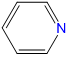, pyridine, abbreviated py pyridine pyridine
CH3CN, acetonitrile, abbreviated MeCN acetonitrile acetonitrile
P3- phosphido phosphido
PH3 phosphine phosphane
PR3 trialkylphosphine (e.g. trimethylphosphine for Me3P) trialkylphosphane (e.g. trimethylphosphane for Me3P)
PAr3 triarylphosphine (e.g. triphenylphosphine for Ph3P) triarylphosphine (e.g. triphenylphosphane for Ph3P), dimethylsulfoxide or DMSO or dmso

dimethylsulfoxide

(sometimes called dimethylsulfoxo but this usage is rare and violates the nomenclature rules for neutral ligands)

(methanesulfinyl)methane or dimethyl(oxido)sulfur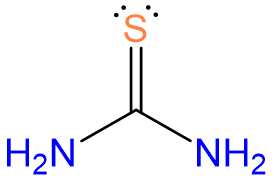, thiourea or tu thiourea thiourea
O2- oxo oxido
OH- hydroxo hydroxido
H2O aqua aqua
S2- sulfo sulfo
HS- hydrosulfido hydrosulfido
RS- alkanethiolate (e.g. ethanthiolate for EtS-) thioalkanoate
H2S hydrogen sulfide hydrogen sulfide
R2S alkylsulfanylalkane (e.g. ethylsulfanylethane for Et2S) dialkyl sulfide
O2 dioxygen dioxygen
O2-, superoxide superoxido dioxido(1-) or superoxido
O22-, peroxide peroxido dioxido(2-) or peroxido
N2 dinitrogen dinitrogen
NO (are always considered neutral for naming purposes) nitrosyl nitrosyl
CO carbonyl carbonyl
CS thiocarbonyl thiocarbonyl
SO, as M-SO sulfino sulfur monoxide-$$\kappa$$S or sulfur monoxide-S
NO2, as M-NO2 nitryl nitrogen dioxide-$$\kappa$$N or nitrogen dioxide-N
CO32- carbonato carbonato
NO2-, as M-NO2 nitro or nitrito-N nitrito-$$\kappa$$N or nitrito-N
NO2-, as M-ONO nitrito or nitrito-O nitrito-$$\kappa$$O or nitrito-O
NO3- nitrato nitrato
SO32- sulfito sulfito
SO42- sulfato sulfato
S2O32-, as M-S-SO2-O- thiosulfato-S thiosulfato-$$\kappa$$S or thiosulfato-S
S2O32-, as M-O-SO2-S- thiosulfato-O thiosulfato-$$\kappa$$O or thiosulfato-O
Table $$\sf{\PageIndex{2}}$$. Common chelating ligands organized by denticity. Most chemists use the common names and abbreviations to describe these ligands.
Common Ligand name IUPAC ligand name abbreviation (if applicable) structure or representative/parent structure (shown in the ionization state in which they bind to a metal)
bidentate ligands
acetylacetonato 2,4-pentanediono acacR-BINAP and S-BINAP R- or S-2,2'-bis(diphenylphosphino)-1,1'-binapthyl BINAP2,2'-bipyridine 2,2'-bipyridine bpy or bipy(binding to the metal occurs through the alkene $$\pi$$ cloud)

dialkyldithiocarbamato dialkylcarbamodithiolato R2NCS2- or dtcdimethylgloximato butanedienedioxime

Hdmg

or DMGdiphenylphosphinoethane

or 1,2-(diphenylphosphino)ethane

Ethane-1,2-diylbis(diphenylphosphane) dppeethylenediamine Ethane-1,2-diamine enethylenedithiolato Ethane-1,2-dithiolato C2H2S22-nacnac N,N'-diphenyl-2,4-pentanediiminato nacnacoxalato oxalato ox1,10-phenanthroline or o-phenanthroline 1,10-phenanthroline phen or o-phenphenylpyridinato

2-phenylpyridinato-C2,N

or 2-phenylpyridinato-$$\kappa$$C2,N

ppytridentate ligands
triazacyclononane 1,3,7-triazacyclononane tacndiethylenetriamine 1,4,7-triazaheptane dienpyrazoylborato

(scorpionate)

hydrotris(pyrazo-1-yl)borato Tpterpyridine

or 2,2';6',2"-terpyridine

12,22:26,32-terpyridine

or 2,6-bis(2-pyridyl)pyridine, tripyridyl, 2,2′:6′,2″-terpyridine

tpy or terpy$$\beta$$, $$\beta$$', $$\beta$$''-triaminotriethylamine $$\beta$$, $$\beta$$', $$\beta$$''-tris(2-aminoethyl)amine tren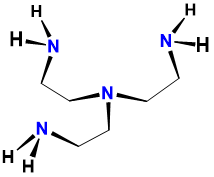triethylenetetramine 1,4,7,10-tetraazadecane triencorroles variable and generally not used cor or Cor12-crown-4 1,4,7,10-tetraoxacyclododecane 12-crown-4tetramethylcyclam 1,4,8,11-tetramethyl-1,4,8,11-tetraazacyclotetradecane TMC or cyclamcyclam 1,4,8,11-tetraazacyclotetradecane cyclamcyclen 1,4,7,10-tetraazacyclododecane cyclen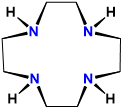tris(2-pyridylmethyl)amine 1-pyridin-2-yl-N,N-bis(pyridin-2-ylmethyl)methanamine tpa or TPAphthalocyanines variable and generally not used variable, usually a modified Pcporphyrins variable and generally not used

variable, usually a modified por, Por, or P

(e.g. TPP = tetraphenylporphyrin)salen 2,2'-ethylenebis(nitrilomethylidene)diphenoxido salen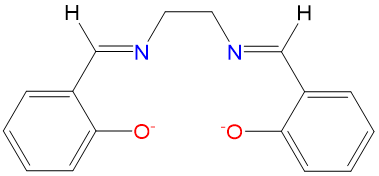15-crown-5 1,4,7,10,13-Pentaoxacyclopentadecane 15-crown-5tetraethylenepentamine 1,4,7,10,13-pentaazatridecane tepa or TEPA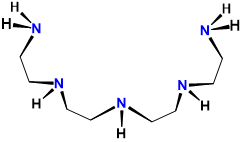18-crown-6 1,4,7,10,13,16-hexaoxacyclooctadecane 18-crown-62,1,1-cryptand 4,7,13,18-Tetraoxa-1,10-diazabicyclo[8.5.5]icosane

2,1,1-crypt

or [2.1.1]-cryptand

kryptofix 211

and variations thereofethylenediaminetetraaceto 2,2′,2″,2‴-(Ethane-1,2-diyldinitrilo)tetraaceto EDTA, edta, Y4-2,2,1-cryptand 4,7,13,16,21-pentaoxa-1,10-diazabicyclo[8.8.5]icosane

2,2,1-crypt

or [2.2.1]-cryptand

kryptofix 221

and variations thereof2,2,2-cryptand 4,7,13,16,21,24-Hexaoxa-1,10-diazabicyclo[8.8.8]hexacosan

2,2,2-crypt

or [2.2.2]-cryptand

kryptofix 222

and variations thereofpentetato acid or diethylenetriaminepentaacetato or DTPA 2-[bis[2-[bis(carboxylatomethyl)amino]ethyl]amino]acetato DTPADOTA or tetraxetan 1,4,7,10-Tetraazacyclododecane-1,4,7,10-tetraacetic acid Dota, DOTA## Rules for Naming Coordination Compounds

As explained above, the name of a coordination compound communicates

• as appropriate, information about isomerism
• systematically listing the ligands in a way that as necessary conveys information about their oxidation state and how they are linked to the metal
• the identity of the metal and its oxidation state
• any counterions present

Before going into these rules it is worth pointing out a few things.

1. It is easiest to learn these rules by starting with one or two of the rules, learning how to apply them, and then adding additional rules one at a time. To that end, instructors who wish to use a more programmed approach may find it convenient to first direct their students to this page which focuses on getting the names of the ligands and metal right, without worrying about isomerism or stereochemistry.

2. The rules also assume some familiarity with common coordination geometries and patterns of isomerism in metal complexes. Thus it might be easiest to learn about common coordination geometries first, followed by common patterns of isomerism in metal complexes before beginning this section. If you decide to dive right in to this section you might find it helpful to know that when applying nomenclature and formula rules most textbooks assume

• complexes in which the metal has a coordination number of six are octahedral
• complexes in which the metal has a coordination number of five are trigonal bipyramidal
• complexes in which PtII , PdII , or RhI, or IrI have a coordination number of four are square planar
• other complexes in which the metal has a coordination number of four are tetrahedral

Like all assumptions these don't always work in real life but they should be good enough to get you through your first inorganic chemistry course.

For the pedants among you, note that the complexes given as examples and in exercises on this page have been selected for pedagogical utility. Although many are well-known compounds, others are hypothetical.

Now on to the rules.

### Rule 1: If ions are present, name the cation first, followed by the anion.

Examples:

K2[PtIICl4] potassium tetrachloroplatinate(2-)

[CoIII(NH3)6](NO3)3 hexaamminecobalt(3+) nitrate

[CoIII(NH3)6][CrIII(C2O4)3] hexaamminecobalt(3+) tris(oxalato)chromium(3-)

### Rule 2: When multiple isomers are possible, designate the particular isomer in italics at the front of the name of each complex

If you have not yet learned about isomerism in coordination compounds skip this rule for now and return to it after you have.

When a complex might exist as one of two stereoisomers, prefixes are commonly used to designate which isomer is present. The most common cases are listed in Table $$\sf{\PageIndex{3}}$$.

Table $$\sf{\PageIndex{3}}$$. Prefixes used to specify isomerism about a metal center when naming and writing coordination compounds' formulae.
Type of isomerism Graphical reminder Prefixes
Geometric, cis- , trans-cis- or trans-
Geometric, fac- /mer-fac- or mer-
Enantiomers, $$\Lambda$$-, $$\Delta$$-$$\Lambda$$- or $$\Delta$$-

Examples of how isomerism about a metal center is designated are given in Scheme $$\sf{\PageIndex{II}}$$.

Scheme $$\sf{\PageIndex{II}}$$. Application of nomenclature rules for stereosimerism about a metal.3There are a number of other cases where it might be advisable to specify the stereochemistry of a complex. These cases involve specifying

• the coordination geometry about a metal center (octahedral, trigonal prismatic, tetrahedral, square planar, etc. )
• the geometry cannot be unambigouously described by a single cis/trans or fac/mer relaionship of ligands

These cases may also be handled by using a designator to specify the coordination geometry and, as necessary, giving the position of ligated atoms in terms of designated numbered positions for that geometry. See the IUPAC red book for details as such cases fall outside the scope of what is normally advisable for an undergraduate course.

### Rule 3: Specify the identity, number, and as appropriate, isomerism of the ligands present in alphabetical order by ligand name.

Before specifying the metal, the ligands are written as prefixes of the metal.

In specifying the ligands several rules are followed.

1. The ligands are written in alphabetical order by the ligand name only; symbols are not considered and prefixes do not count in determining alphabetical order.

Example: In the name of the complex ion [Co(NH3)5Cl]2+, pentamminechlorocobalt(II), the ammine ligand is named before the chloro ligand because the order is alphabetical by the ligand name by virtue of which ammine comes before chloro.

1. Prefixes are used to indicate the number of each ligand present. Specifically, di-, tri-, tetra- , penta-, hexa-, etc. prefixes are used to indicate multiple ligands of the same type EXCEPT when the ligand is polydentate or its name already has a di-, tri-, tetra- etc. In that case bis-,tris-, tetrakis-, etc. are used instead. These prefix rules are summarized in Table $$\sf{\PageIndex{3}}$$.
Table $$\sf{\PageIndex{3}}$$. Prefixes used to specify the number of a given ligand present.
Number of identical ligands prefix used when the ligand name is simple prefix used when the ligand is polydentate or its name already has a di-, tri-, tetra- etc.
2 di- bis-
3 tri- tris-
4 tetra- tetrakis-
5 penta- pentakis-
6 hexa- hexakis-
7 hepta- heptakis-
8 octa- octakis-
9 nona- nonakis-
10 deca- decakis-

An example of the application of the prefix rule is given in Scheme $$\sf{\PageIndex{III}}$$.

Scheme $$\sf{\PageIndex{III}}$$. Example of the use of prefixes to specify the number of ligands of each type in a complex.31. Ligand names are based on their charge.
• Neutral and cationic ligand names are the same as the names of their neutral compounds with two caveats:
1. names that involve spaces should either be put in parentheses or the spaces should be eliminated (preferred)

Example: cis-dichlorobis(dimethyl sulfoxide)platinum(II) or cis-dichlorobis(dimethylsulfoxide)platinum(II)

1. A few ligands are given common names.
• H2O = aqua
• NH3 = ammine (notice that there are two n's)
• CO = carbonyl
• CS = thiocarbonyl
• NO = nitrosyl
• For anionic ligands, the vowel at the end of their anion names is changed to an -”o”

Examples: Cl- = chloro, NH2- = amido, N3- = azido

Caveat: some anionic ligands have common names that may also be used

Examples:

I- = iodo or iodino

CN- = cyano or cyanido

O2- = oxo or oxido

The IUPAC and common names of many ligands are given in Tables $$\sf{\PageIndex{1}}$$. and $$\sf{\PageIndex{2}}$$.

1. When an ambidentate ligand is present, the atom through which it is bound to the metal is indicated by giving either its element symbol or a $$\kappa$$ and its element symbol in italics after the ligand name.

Example:

M-SCN is thiocyanato-S or thiocyanato-$$\kappa$$S

M-NCS = thiocyanato-N or thiocyanato-$$\kappa$$N

The use of $$\kappa$$ and an element symbol to indicate how a ligand and metal are linked is called a k-term. More complex k-terms might also involve specifying the atoms by number, though their use is outside the scope of this text.

1. As appropriate, additional information about the way a ligand is bound to the metal center and/or its stereochemistry is specified using a prefix. The prefixes to provide linkage and stereochemistry for ligands are given in Table $$\sf{\PageIndex{4}}$$.
Table $$\sf{\PageIndex{4}}$$. Prefixes used to specify ligands' isomerism when naming and writing coordination compounds' formulae. Some of these types of isomerism will be discussed in later pages.
Type of isomerism Graphical reminder Prefixes
when a multidentate ligand binds through less than the full number of atoms$$\kappa$$n where n is the number of attached atoms; used when the attached atoms are not directly connected by a chemical bond. The metal-ligand bonding usually involves $$\sigma$$-type coordination.
hapticity$$\eta$$n where n is the number of attached atoms; used when the coordinated atoms are all connected by bonds. Usually the metal-ligand bonding involves $$\pi$$-type coordination.

In speech, $$\eta^1$$=monohapto; $$\eta^2$$=dihapto; $$\eta^3$$=trihapto, etc.

bridging ligands$$\mu$$n where n is the number of atoms bridged. The number n is usually omitted when n =2.
chelating ligand ring twist$$\lambda$$- or $$\delta$$-

A example showing how the nomenclature rule is applied to a ligand that can have two coordination modes is given in Scheme $$\sf{\PageIndex{IV}}$$.

Scheme $$\sf{\PageIndex{IV}}$$. Use of the $$\kappa$$ notation to specify the number of attached groups in a multidentate ligand.Scheme $$\sf{\PageIndex{V}}$$. The names of the complexes follow the rules described in 9.2.6: multinuclear coordination complexes.

Scheme $$\sf{\PageIndex{V}}$$. Use of the $$\mu$$ notation to specify bridging ligands in metal complexes.31. If desired, parentheses may be used to delineate a ligand name to make it easier to identify in the name of the complex. This can be particularly helpful when the entire name contains a lot of information to keep track of. An example is given in Scheme $$\sf{\PageIndex{VI}}$$.

Scheme $$\sf{\PageIndex{VI}}$$. When naming the complex shown, cis-diaquabis(ethylenediamine)chromium(III) nitrate is easier to read than cis-diaquabisethylenediaminechromium(III) nitrate.### Rule 4: Specify the identity of the metal

• In neutral and cationic complexes the metal's name is used directly

- e.g. as in hexammineruthenium(III) for [Ru(NH3)6]3+

• In anionic complexes, -ate replaces -ium, -en, or –ese or adds to the metal name,

e.g., as in hexachloromanganate(IV) for MnCl62-

• In anionic complexes of some metals a Latin-derived name is used instead of the element's English name. These names are given in Table $$\sf{\PageIndex{5}}$$.
Table $$\sf{\PageIndex{5}}$$. Latin terms for select metal Ions. Redrawn from this page describing the nomenclature of coordination complexes.
Transition Metal Latin
Copper Cuprate
Gold Aurate
Iron Ferrate
Silver Argentate
Tin Stannate

An example of the application of the metal naming rules is given in Scheme \sf{\PageIndex{VII}}\).

Scheme $$\sf{\PageIndex{VII}}$$. Example of the application of the metal specification rules to a cationic and an anionic platinum complex.3### Rule 5: Specify the oxidation state of the metal.

Two different systems are used to specify the oxidation state of the metal.

1. In the Stock system the metal's oxidation state is indicated in Roman numerals after the metal name.

Examples:

[CoCl(NH3)5]Cl2 = pentamminechlorocobalt(III) chloride

[PtBr2(bpy)] = dibromobipyridineplatinium(II)

K[Ag(SCN)2] = potassium di-S-thiocyanatoargentate(I)

1. In the Ewing-Bassett system the charge on the complex is specified in Arabic numerals after the complex name. This provides a way of specifying a complex even when the oxidation state of the metal isn't known and, in cases where it is known, the value of the metal's oxidation state may be inferred from the complex ion's charge.

[CoCl(NH3)5]Cl2 = pentamminechlorocobalt(2+) chloride

[PtBr2(bpy)] = dibromobipyridineplatinium(0)

K[Ag(SCN)2] = potassium di-S-thiocyanatoargentate(1-)

### Summary of rules for naming coordination complexes.

A graphical summary of the rules for naming complexes along with a few examples that you can use to review the nomenclature rules is given in Figure $$\sf{\PageIndex{1}}$$. This work by Stephen Contakes is licensed under a Creative Commons Attribution 4.0 International License.Figure $$\sf{\PageIndex{1}}$$. Summary of nomenclature rules for coordination complexes along with a few examples of their application.

When learning chemical nomenclature, practice makes perfect. The following examples and exercises are provided to give you this practice. Additional examples and exercises on the https://chem.libretexts.org/ site include a set of simple examples with explained solutions, a set of simple exercises with solutions, and a set of more challenging exercises without solutions.

##### Exercise $$\PageIndex{2}$$. Assigning metal oxidation states in a complex

In order to name a complex in the Stock system it is necessary to assign a formal oxidation state to the metal. For this reason it is important to be able to assign the oxidation state of a metal in a complex. Fortunately, this is easy to do if you remember

1. The sum of all atoms' oxidation states will equal the overall charge on the complex
2. When determining the metal's oxidation state the ligands can be treated as having an oxidation state equal to their charge - i.e., the charge they possess in the form in which they coordinate the metal, so if they need to lose a proton to bind, don't forget to account for that.

Given the above, assign the oxidation state of the metal in the following real and hypothetical complexes.

1. K3[Fe(CN)6]
2. [CoCl(NH3)5](NO3)2
3. K2[PtCl4]
4. [MnCl(por)]
5. [Ru(bpy)3]Cl2
6. [PdCl2(dppe)]
7. [Mn(en)2(SCN)2]

This contains [Fe(CN)6]3-; so O.S.Fe + 6 x (-1) (for CN-) = -3 (the complex's charge) so O.S.Fe = +3 or Fe3+.

This contains [CoCl(NH3)5]2+; so O.S.Co + 1 x (-1) (for Cl-) + 0 x 5 (for NH3) = +2 (the complex's charge) so O.S.Co = +3 or Co3+.

This contains 2K+ and [PtCl4]2-; so O.S.Pt + 4 x (-1) (for Cl-) = -2 (the complex's charge) so O.S.Pt = +2 or Pt2+.

O.S.Pt + 1 x (-2) (for por; see table 9.2.2) + 1 x (-1) (for Cl-) = +0 (the complex's charge) so O.S.Mn = +3 or Mn3+.

The complex is [Ru(bpy)3]2+ so O.S.Ru + 0 x 3 (for bpy) = +2 (the complex's charge) so O.S.Ru = +2 or Ru2+.

O.S.Pd + 2 x (-1) (for Cl-) + 0 x 3 (for dppe) = +0 (the complex's charge) so O.S.Pd = +2 or Pd2+.

O.S.Mn + (2 x 0) (for en) + 2 x (-1) (for SCN-) = +0 (the complex's charge) so O.S.Mn = +2 or Mn2+.

##### Exercise $$\PageIndex{3}$$: Simple Nomenclature Problems.

Name the following compounds in both the Stock and Ewing-Bassett systems:

1. [Ru(NH3)6](NO3)3
2. K2[PtCl4]
3. K[Ag(CN)2]
4. Cs[CuBrCl2F]
5. [Cu(acac)2]
Complex Stock system name Ewing-Bassett System name
a [Ru(NH3)6](NO3)3 hexammineruthenium(III) nitrate hexammineruthenium(3+) nitrate
b K2[PtCl4] potassium tetrachloroplatinate(II) potassium tetrachloroplatinate(2-)
c K[Ag(CN)2] potassium dicyanoargentate(I) potassium dicyanoargentate(1-)
d Cs2[CuBrCl2F] cesium bromodichlorofluorocuprate(II) cesium bromodichlorofluorocuprate(2-)
e [Cu(acac)2] bis(acetylacetonato)copper(II) bis(acetylacetonato)copper(0)
##### Exercise $$\PageIndex{4}$$: More simple nomenclature problems.

Name the following compounds and ions in both the Stock and Ewing-Bassett systems.

1. Cu(OH)4-
2. [AuXe4]2+
3. AuCl4-
4. Fe(CN)63-
5. K4[Fe(CN)6]
6. trans-[Cu(en)2(NO2)2] (the N is bound to Cu)
7. cis-IrCl2(CO)(PPh3) (ignore stereochemistry)
8. IrCl(PPh3)3
Compound Stock System Name Ewing-Bassett System Name
a Cu(OH)4- tetrahydroxidocuprate(III) or tetrahydroxidocuprate(III) tetrahydroxidocuprate(1-) or tetrahydroxidocuprate(1-)
b [AuXe4]2+ tetraxenongold(II) tetraxenongold(2+)
c AuCl4- tetrachloroaurate(III) tetrachloroaurate(1-)
d Fe(CN)63- hexacyanoferrate(III) or hexacyanidoferrrate(III) hexacyanoferrate(3-) or hexacyanidoferrate(3-)
e K4[Fe(CN)6] potassium hexacyanoferrate(II) or potassium hexacyanidoferrrate(II) potassium hexacyanoferrate(4-) or potassium hexacyanidoferrrate(4-)
f trans-[Cu(en)2(NO2)2] (the N is bound to Cu) bis(ethylenediamine)bisnitrocopper(II) or bis(ethylenediamine)bis(nitrito-$$\kappa$$N)copper(II) bis(ethylenediamine)bisnitrocopper(0) or bis(ethylenediamine)bis(nitrito-$$\kappa$$N)copper(0)
g cis-IrCl2(CO)(PPh3)

cis-dichlorocarbonyltriphenylphosphineiridium(I)

or cis-dichloro(carbonyl)(triphenylphosphine)iridium(I)

cis-dichlorocarbonyltriphenylphosphineiridium(0)

or cis-dichloro(carbonyl)(triphenylphosphine)iridium(0)

h IrCl(PPh3)3 chlorotris(triphenylphosphine)iridium(I) chlorotris(triphenylphosphine)iridium(0)
##### Exercise $$\PageIndex{5}$$: Even more simple nomenclature problems.

Name the following compounds and ions in both the Stock and Ewing-Bassett systems. Ignore prefixes for designating isomers if you haven't learned about those.

1. Fe(acac)3
2. K2[CuBr4]
3. ReH9
4. [Ag(NH­3)2]BF4
5. [Ag(NH­3)2][Ag(CN)2]
6. [Ni(CN)4]2-
7. [Co(N3)(NH­3)5]SO4
8. [CoBrCl(H2O)(NH3)]I (ignore stereochemistry)
Complex Stock system name Ewing-Bassett System name
a Fe(acac)3 tris(acetoacetato)iron(III) tris(acetoacetato)iron(0)
b Na2[CuBr4] sodium tetrabromocuprate(II) sodium tetrabromocuprate(2-)
c [Co(NH3)6][Co(ox)3] hexamminecobalt(III) tris(oxalato)cobalt(III) hexamminecobalt(3+) tris(oxalato)cobalt(3-)
d [Ag(NH­3)2]BF4 diamminesilver(I) tetrafluoroborate diamminesilver(1+) tetrafluoroborate
e [Ag(NH­3)2][Ag(CN)2]

diamminesilver(I) dicyanoargentate(I)

or diamminesilver(I) dicyanidoargentate(I)

diamminesilver(1+) dicyanoargentate(1-)

or diamminesilver(1+) dicyanidoargentate(1-)

f [Ni(CN)4]2- tetracyanonickelate(II) or tetracyanonickelate(II) ion tetracyanonickelate(2-) or tetracyanonickelate(2-) ion
g [Co(N3)(NH­3)5]SO4 pentammineazidocobalt(III) sulfate pentammineazidocobalt(2+) sulfate
h

[CoBrCl(H2O)(NH3)]I

(ignore stereochemistry)

ammineaquabromochlorocobalt(III) iodide ammineaquabromochlorocobalt(1+) iodide
##### Exercise $$\PageIndex{6}$$: Nomenclature problems, some of which involve consideration of isomerism.

Name the following compounds and ions in both the Stock and Ewing-Bassett systems. Ignore prefixes for designating isomers if you haven't learned about those.

1. trans-[Cu(dppe)2(NO2)2] (the N is bound to Cu)
2. [Pd(en)2(SCN)2], with the thiocyanates bound Pd-SCN
3. [Mn(CO)6]BPh4 (BPh4 = tetraphenylborate)
4. Rb[AgF4]
5. K2ReH9
6. K3CrCl6
7. [Ru(H2O)6]Cl2
8. [cis-Fe(CO)4I2]
9. K2[trans-Fe(CN)4(CO)2]
10. [cis-MnCl(H2O)4(NH3)](NO3)
11. K3[fac-RuCl3(PMe3)3]
Complex Stock system name Ewing-Bassett System name
a trans-[Cu(dppe)2(NO2)2] trans-bis(diphenylphosphinoethane)bisnitrocopper(II) or trans-bis(diphenylphosphinoethane)bis(nitrito-$$\kappa$$N)copper(II) trans-bis(diphenylphosphinoethane)bisnitrocopper(0) or trans-bis(diphenylphosphino)ethanebis(nitrito-$$\kappa$$N)copper(0)
b [Pd(en)2(SCN)2], with the thiocyanates bound Pd-SCN

or bis(ethylenediamine)bis(thiocyanato-$$\kappa$$S)palladium(II)

or bis(ethylenediamine)bis(thiocyanato-$$\kappa$$S)palladium(0)

c [Mn(CO)6]BPh4 hexacarbonylmanganese(I) tetraphenylborate hexacarbonylmanganese(1+) tetraphenylborate
d Rb[AgF4] rubidium tetrafluoroargentate(III) rubidium tetrafluoroargentate(1-)
e K2ReH9 potassium nonahydridorhenium(VII) potassium nonahydridorhenium(2-)
f K3CrCl6 or K3[CrCl6]

potassium hexachlorochromium(III) or

potassium hexachloridochromium(III)

potassium hexachlorochromium(3-) or

potassium hexachloridochromium(3-)

g [Ru(H2O)6]Cl2 hexaaquaruthenium(II) chloride hexaaquaruthenium(2+) chloride
h [cis-Fe(CO)4I2] cis-tetracarbonyldiiodoiron(II) cis-tetracarbonyldiiodoiron(0)
i K2[trans-Fe(CN)4(CO)2] potassium trans-dicarbonyltetracyanoferrate(II) potassium trans-dicarbonyltetracyanoferrate(2-)
j [cis-MnCl(H2O)4(NH3)](NO3) cis-amminetetraaquachloromanganese(0) nitrate cis-amminetetraaquachloromanganese(1+) nitrate
k K[fac-RuCl3(PMe3)3] potassium fac-trichlorotris(triphenylphosphine)ruthenium(II) potassium fac-trichlorotris(triphenylphosphine)ruthenium(1-)
##### Exercise $$\PageIndex{7}$$

Name the compounds and ions below using both the Stock and Ewing-Bassett systems. Ignore prefixes for designating isomers if you haven't learned about those.# Structure Stock system name Ewing-Bassett system name
1cis-tetraacetonitriledicyanoiron(II)

or cis-tetraacetonitriledicyanidoiron(II)

cis-tetraacetonitriledicyanoiron(0)

or cis-tetraacetonitriledicyanidoiron(0)

2trans-tetraacetonitriledicyanoiron(II)

or trans-tetraacetonitriledicyanidoiron(II)

trans-tetraacetonitriledicyanoiron(0)

or trans-tetraacetonitriledicyanidoiron(0)

3trans-bromochlorobis(ethylenediamine)iron(III)

or trans-bromidochloridobis(ethylenediamine)iron(III)

trans-bromochlorobis(ethylenediamine)iron(1+)

or trans-bromidochloridobis(ethylenediamine)iron(1+)

4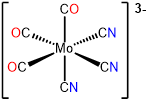fac-tricarbonyltricyanomolybdate(0)

or fac-tricarbonyltricyanidomolybdate(0)

fac-tricarbonyltricyanomolybdate(3-)

or fac-tricarbonyltricyanidomolybdate(3-)

5mer-tricarbonyltricyanomolybdate(0)

or mer-tricarbonyltricyanidomolybdate(0)

mer-tricarbonyltricyanomolybdate(3-)

or mer-tricarbonyltricyanidomolybdate(3-)

6pentamminenitrito-N-cobalt(III),

pentamminenitrito-$$\kappa$$N-cobalt(III),

or pentamminenitrocobalt(III)

pentamminenitrito-N-cobalt(2+),

pentamminenitrito-$$\kappa$$N-cobalt(2+),

or pentamminenitrocobalt(2+)

7pentamminenitrito-O-cobalt(2+),

pentamminenitrito-$$\kappa$$O-cobalt(2+),

or pentamminenitritocobalt(2+)

pentamminenitrito-O-cobalt(2+),

pentamminenitrito-$$\kappa$$O-cobalt(2+),

or pentamminenitritocobalt(2+)

##### Exercise $$\PageIndex{8}$$

Draw structural formulae for the following compounds and ions. You may assume that

• complexes in which the metal has a coordination number of six are octahedral
• complexes in which the metal has a coordination number of five are trigonal bipyramidal
• complexes in which PtII , PdII , or RhI, or IrI have a coordination number of four are square planar
• other complexes in which the metal has a coordination number of four will be tetrahedral
1. (2,2'-bipyridine)tetracyanoruthenium(2-)
2. sodium tetrachloroaluminate (note that since Al is a main group metal with a generally fixed oxidation state no oxidation state is given)
3. pentaamminechlorocobalt(2+) sulfate
4. carbonylhydridotris(triphenylphosphine)rhodium(I) (the ligands in this complex occupy sterically preferred positions)
5. bromotrichlorocobaltate(III)
6. hexaaquacopper(2+) sulfate
7. sodium tris(oxalato)cobalt(III)
8. fac-(1,10-phenanthroline)tricarbonylchlororhenium(I)
9. mer-triaquatrichlorochromium(III)
10. trans-dichlorobis(ethylenediamine)platinum(IV)
abcde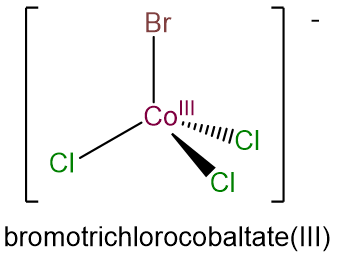f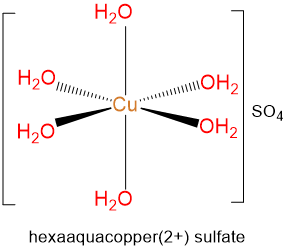gh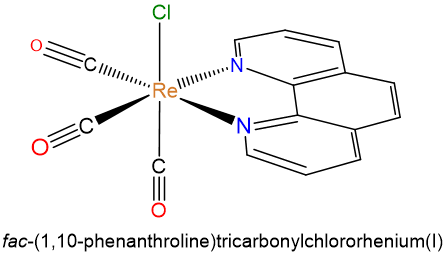ij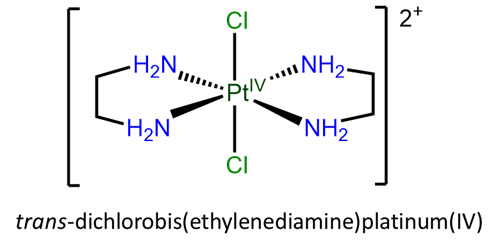##### Exercise $$\PageIndex{9}$$.

The name of the structure named tris(tetraammine-$$\mu$$-dihydroxocobalt)cobalt(6+) in Scheme $$\sf{\PageIndex{III}}$$ (reproduced below) is incomplete. Give the complete name of the structure in both the Stock and Ewing-Basset systems.$$\Delta$$-tris(tetraammine-$$\mu$$-dihydroxocobalt)cobalt(6+) or $$\Delta$$-tris(tetraammine-$$\mu$$-dihydroxocobalt(III)cobalt(III)

## Rules for writing the formulae of coordination compounds

The rules for writing the formulae of coordination compounds follow the same convention used to specify their names. However, according to a myth that has been widely propagated on educational websites, the charge need not be listed. As organized predictable formulae can be easier to read and understand than haphazardly written ones, the rules for writing the formulae of coordination compounds have value. To support those who wish to employ them they are given in this section. The rules as given here are adapted from a summary by Robert Lancashire.

1. If there are multiple ions present list the cations before anions.
2. Enclose all the constituents of each complex ion in square brackets.
3. For each complex ion,
• Give the central metal atom first.
• Then ligands next, listed in alphabetical order, ignoring prefixes according to the first letters in the ligand's symbol as written. This is true regardless of whether the symbol is an element symbol (like C, N, O, etc.) or a symbol for the ligand name (bpy, en, MeCN, etc.). Contrary to widely-circulated myths, the ligand's charge does not matter.4
• The formulae or abbreviations (e.g. en) for all polyatomic ligands should be enclosed in ordinary parentheses.
• As appropriate, use italicized atom symbols to indicate linkage isomerism and prefixes such as cis-, trans-, fac-, mer-, $$\Lambda$$-, $$\Delta$$-, κn, ηn , μn, $$\lambda$$-, or $$\delta$$- to indicate stereochemistry.
• When a ligand is bound to a metal through a particular atom, preferably place that atom closest to the metal - e.g., [Fe(CN)6]3- not [Fe(NC)6]3- (Note: this rule is used primarily for ambidentate ligands; although IUPAC recommends that aqua ligands be written as OH2 when the O would be closest to its coordinated metal, they are still usually written as H2O).

These rules are graphically summarized in Figure $$\sf{\PageIndex{2}}$$.Figure $$\sf{\PageIndex{2}}$$. Summary of the rules for writing the formula of a coordination complexes along with a few examples of their application. This work by Stephen Contakes is licensed under a Creative Commons Attribution 4.0 International License.
##### Exercise $$\PageIndex{10}$$

Give the formulae of the following complexes..##### Exercise $$\PageIndex{11}$$

Write formulae for each of the following compounds and ions. When multidentate ligands are present use suitable abbreviations.

1. pentaamminechlorocobalt(2+) sulfate
2. $$\Delta$$-diamminebis(oxalato)manganate(III)
3. trans-tetraacetonitriledicyanoiron(II)
4. tricarbonyldichlorobis(triphenylphosphine)molybdenuma.

[CoCl(NH3)5](SO4)

b.

$$\Delta$$-[Mn(NH3)2(ox)2]-

c.

trans-[Fe(CN)2(NCMe)4]

d.

[MoCl2(CO)3(PPh3)] or MoCl2(CO)3(PPh3)

e.

mer-[CrBrCl(H2O)3I]

f.

[Co(O2)py(salen)]

g.

[Fe(NO)2(SEt)2]-

h.

[MnCl(por)]- or [MnCl(porphyrin)]-

i.

[Ni(DMG)2] or [Ni(Hdmg)2]

j.

potassium trans-[Fe(CN)2(CO)4]

k.

trans-[CuCl(H2O)4(NH3)]SO4

l.

trans-[PtCl2(en)2]2+

m.

cis-[CoBrCl(NH3)4]SO4

n.

K2[Fe(CN)5NO]

## Multinuclear coordination complexes

Multinuclear coordination complexes contain multiple metals connected by one or more bridging ligands. The structures of bridging complexes can usually be inferred from their $$\mu$$-tagged ligands in their names and formulae. For the benefit of instructors who wish to have their students name multinuclear complexes, the rules are presented below.

Multinuclear complexes are named differently depending on whether the groups on either side of the bridging ligands are identical or different, as shown in Scheme $$\sf{\PageIndex{VIII}}$$.

Scheme $$\sf{\PageIndex{VIII}}$$. Multinuclear coordination complexes may be named differently depending on whether the groups on either side of the bridging ligands are the same or different. The groups are the same if the metal, ligands, and ligand arrangement are identical. This is true in A but in B the metals differ, in C the ligands differ, and in D both the metal and ligands differ.### Naming multinuclear complexes

Let's look at the rules for naming symmetric and asymmetric multinuclear complexes.

#### Application of the IUPAC system and variants thereof to symmetric complexes

The IUPAC naming system helpfully avoids the sort of ambiguities and ad hoc choices involved in most textbook-level nomenclature systems for multimetallic complexes. Unfortunately, it is correspondingly difficult for beginners to employ. Thus it will only be applied in depth to the case of symmetric complexes. Symmetric complexes are particularly easy to name in the IUPAC system and several of its variants that find common use. In these

1. The ligands are given in alphabetical order. When there are bridging and non-bridging ligands of the same type the bridging ligands are given first. When there are multiple bridging ligands of the same type but which use different bridging modes (e.g., $$\mu$$4-, $$\mu$$3-, $$\mu$$2-), the bridging ligands are specified in decreasing order of bridging multiplicity, e.g., $$\mu$$3 -sulfido-di-$$\mu$$-sulfido.
2. The groups bridged are given afterwards using names that follow the ordinary rules. Generally this involves either
1. naming all the ligands followed by all the metals, in both cases using prefixes to indicate the number of each, or
2. naming each group of atoms individually as in the less formal naming system, using prefixes to indicate the number of ligands (this is an unofficial variant of the IUPAC system that some textbook authors seem to prefer).

These two rules are sufficient to describe simple symmetric bridging complexes.

Example: The compound in Scheme $$\sf{\PageIndex{VIIIA}}$$may be named

[$$\mu$$-amido-$$\mu$$-hydroxo-octaamminedichromium(4+)] ion

or

[$$\mu$$-amido-$$\mu$$-hydroxo-bis(tetraamminechromium(III))] ion

or

[$$\mu$$-amido-$$\mu$$-hydroxo-bis(tetraamminechromium)(4+)] ion

Example: The complex5may be named [tri-$$\mu$$-carbonyl-bis(tricarbonyliron)(0)], [tri-$$\mu$$-carbonyl-bis(tricarbonyliron(0))], or [tri-$$\mu$$-carbonyl-hexacarbonyldiiron)(0)]

Example: The complexmay be named di-$$\mu$$-chlorido-tetrachloridodicopper(II), di-$$\mu$$-chlorido-bis(dichlorocopper(II)), or di-$$\mu$$-chlorido-bis(dichlorocopper)(0)

#### A note on the application of the IUPAC system and variants thereof to asymmetric complexes

For a symmetrical complex like the [Cu2Cl4($$\mu$$-Cl)2] considered above it is enough to specify the existence of the two bridging and four terminal chloro ligands; there is no need to number the chloro ligands or to specify exactly which ones are involved in bridging or to clarify that the bridging involves $$\kappa$$2 coordination of the chloro ligands. In cases where the two metal centers or the chloro ligands differ it is necessary to specify the exact ligands and metals involved and how they are connected.

For example, a more extensive IUPAC name for [Cu2Cl4($$\mu$$-Cl)2] in which the chloro ligands are individually and more completely specified would read di-$$\mu$$2-chlorido-tetrachlorido-1$$\kappa$$2Cl,2$$\kappa$$2Cl-dicopper(II).

Even more extensive systems would involve numbering the metals and specifying how they are connected together too. The details of how this is done are typically beyond the level of most undergraduate and graduate courses in inorganic chemistry. Those who want to know the details should consult the red book. In many cases it is sufficient to reserve the use of formal IUPAC names for use in publications and to employ a convenient shorthand naming system for everyday use. An example of one type of system that is sometimes employed is given next.

#### Unofficial but commonly used methods applicable to complexes in which the groups bridged are identical or different.

It can be quite complicated to use the IUPAC system to name asymmetric multinuclear coordination complexes. Fortunately it is rarely necessary and there are a variety of simpler somewhat ad hoc methods that work well for such cases. Since these methods are commonly used by textbooks and inorganic instructors it is likely worth your while to learn about their general features. In these methods

1. A multinuclear complex is named as a derivative of one of the metal centers and the other metal centers and their ligands are treated as ligands to the prioritized metal center.
2. Unfortunately, most of the time the choice of which metals are part of the ligands and which one is central is made haphazardly. In other words, there is no agreed upon system for assigning which metal center is the central metal and which should be regarded as part of the ligands around it. One way to be more systematic about the selection of the central and ligand-embedded metals is to assign the central metal as the metal of highest priority in the IUPAC priority rules. In the IUPAC priority rules, the central metal is the highest priority metal alphabetically by name; then if there is a tie the ligands on each tied metal center are ranked alphabetically and the tied metal center with the highest priority (or most highest priority) ligands wins.
3. The metal centers not chosen as the primary metal center and all ligands around them, including those bridging to the winning metal center, are then treated as a single ligand that coordinates the winning metal center.
4. When naming the ligands and the overall complex, again there is much that is haphazard. However, in the best systems each coordination sphere is named using the same rules as for mononuclear complexes - i.e., the ligands are given in alphabetical order, etc.
• This is true of any metal center-containing ligands.
• When there is a bridging and non-bridging ligand of the same type, the bridging ligands are given first. When there are multiple bridging ligands of the same type but which use different bridging modes (e.g. $$\mu$$4-, $$\mu$$3-, $$\mu$$2-), the ligands are specified in decreasing order of bridging multiplicity, e.g. $$\mu$$3 -sulfido-di-$$\mu$$-sulfido.

Let's apply these rules to the examples in Scheme $$\sf{\PageIndex{VIII}}$$.

Compound A:Using hydroxo for the OH-, it may be named

[($$\mu$$-amido-tetraammine-$$\mu$$-hydroxochromium(III))tetraamminechromium(III)] ion

or

[($$\mu$$-amido-tetraammine-$$\mu$$-hydroxochromium)chromium(4+)] ion

Compound B:Using hydroxo for the OH-, it may be named

[(pentaammine-$$\mu$$-hydroxocobalt(III))tetraamminechromium(III)] ion

or

[(pentaammine-$$\mu$$-hydroxocobalt)tetraamminechromium(5+)] ion

Compound C:Using hydroxo for OH-, it may be named

[pentaammine(pentachloro-$$\mu$$-hydroxocobalt(III))cobalt(III)]

or

[pentaammine(pentachloro-$$\mu$$-hydroxocobalt)cobalt](0)]

Compound D:Using hydroxo for OH- and chloro for Cl-, it may be named

[pentaammine(pentachloro-$$\mu$$-hydroxocobalt(III))chromate(III)] ion

or

[(tetrammine-$$\mu$$-ammine-$$\mu$$-hydroxocobalt)tetrachlorochromate](1-)] ion

As can be seen from the examples above this system gives serviceable names for multimetallic complexes but those names are not the IUPAC names and so should not be used to describe complexes outside of pedagogical and informal settings.

### Writing and interpreting formulae for multinuclear complexes

The rules for writing formulae for multinuclear complexes are the same as for mononuclear ones with two added details:

1. Write bridging ligands after nonbridging ligands of the same type. For example, Cu2Cl6 should be written as [Cu2Cl4-($$\mu$$-Cl)2]
2. However, you may ignore that and other rules if you find it helpful to keep groups of metals and ligands together in a way that better conveys how the atoms are connected. Just as the structure of ethane may be more clearly conveyed as H3CCH3 instead of CH3CH3, the complex [Cu2Cl4($$\mu$$-Cl)2] may be written as [(Cl2Cu)($$\mu$$-Cl)2(CuCl2)].

Other Examples:

For dichromate write, [Cr2O6($$\mu$$-O)]2- or [O5Cr-$$\mu$$-O-CrO5]2-

For compound C of Scheme $$\sf{\PageIndex{VIII}}$$.write [Co2Cl5(NH3)5($$\mu$$-OH)] or, even better, [(H3N)5Co($$\mu$$-OH)CoCl5].

##### Exercise $$\PageIndex{10}$$

Write reasonable formulae for complexes A, B, and D in Scheme $$\sf{\PageIndex{VIII}}$$, which for convenience is reproduced below.[Cr2(NH3)8-$$\mu$$(NH2)-$$\mu$$(OH)]4+ or [(H3N)4CrIII-$$\mu$$(NH2)-$$\mu$$(OH)-CrII(NH3)4] and variants thereof involving writing the H3N as NH3, $$\mu$$ as $$\mu_2$$, etc.

[CoCr(NH3)10-$$\mu$$(OH)]5+ or, better, [(H3N)5CoIII-$$\mu$$(OH)-CrIII​​​​​​(NH3)5]5+

This was already done as an example above, [Co2Cl5(NH3)5-$$\mu$$(OH)] or, better, [(NH3)5CoIII-$$\mu$$(OH)-CoIIICl5] and variants thereof

[CoCrCl4(NH3)4-$$\mu$$(NH2)-$$\mu$$(OH)] or, better, [(H3N)4CoIII-$$\mu$$(NH2)-$$\mu$$(OH)-CrIICl4]

## References

1. International Union of Pure and Applied Chemistry Nomenclature of Inorganic Chemistry Cambridge, UK, 2005.

2. The structure and name is taken from Choudhury, S. B.; Allan, C. B.; Maroney, M.; Wodward, A. D.; Lucas, C. R. Inorg. Synth. 1998, 32, 98-107.

4. There is a widely-circulated myth that anionic ligands should be named before neutral ones. This myth is false. According to the IUPAC red book (bolding mine):

IR-2.15.3.4: Ordering ligands in formulae and names in formulae of coordination compounds,

The formulae or abbreviations representing the ligands are cited in alphabetical order as the general rule. Bridging ligands are cited immediately after terminal ligands of the same kind, if any, and in increasing order of bridging multiplicity. (See also Sections IR-9.2.3 and IR-9.2.5.)

IR-9.2.3.1 Sequence of symbols within the coordination formula

(i) The central atom symbol(s) is (are) listed first.

(ii) The ligand symbols (line formulae, abbreviations or acronyms) are then listed in alphabetical order (see Section IR-4.4.2.2).5 Thus, CH3CN, MeCN and NCMe would be ordered under C, M and N respectively, and CO precedes Cl because single letter symbols precede two letter symbols. The placement of the ligand in the list does not depend on the charge of the ligand.

(iii) More information is conveyed by formulae that show ligands with the donor atom nearest the central atom; this procedure is recommended wherever possible, even for coordinated water.

5. Technically organometallic complexes are named according to slightly different rules but the coordination compound naming system works here. Note that this complex was once thought to possess an Fe-Fe bond, in accordance with the predictions of the 18-electron rule. Consequently, older sources often give an [Fe-Fe] after the name to reflect the presence of that bond. However, as the complex is no longer thought to possess an Fe-Fe bond it is omitted here.

9.3: Nomenclature and Ligands is shared under a not declared license and was authored, remixed, and/or curated by Stephen M. Contakes.